Definitions of Square Dance Calls and Concepts
Big Block CONCEPT [C3A]Index -->  Plus  |  A1  |  A2  |  C1  |  C2  |  C3A  |  C3B  |  C4  |  NOL  |
Definitions (Text Only) -->  Plus  |  A1  |  A2  |  C1  |  C2  |  C3A  |  C3B  |  C4  |  NOL  |
 Find call:

 \$B8@8l(BInterlocked Blocks, Butterfly, "O" \$B\$^\$?\$OB>\$NE,@Z\$J(B formation \$B\$+\$i!%(B

\$BM?\$(\$i\$l\$?%3!<%k\$r(B Distorted Parallel Lines \$B\$GF0\$-\$^\$9!%%3!<%k\$N=*\$o\$j\$G(B, \$B3F!9\$N?M\$O%*%j%8%J%k\$N(B formation \$B\$N0LCV(B (\$BLu

Big Block CONCEPT: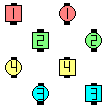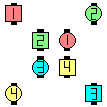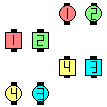\$B\$3\$3\$+\$i(B... \$B\$^\$?\$O\$3\$3\$+\$i(B... \$B\$^\$?\$O\$3\$3\$+\$i(B...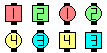\$B\$3\$N(B formation \$B\$+\$iF0\$/\$H9M\$(\$^\$9(B

Big Block In Roll Circulate: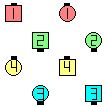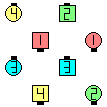Big Block In Roll Circulate\$B\$NA0(B \$B8e(B

Big Block Trade Circulate: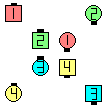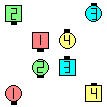Big Block Trade Circulate\$B\$NA0(B \$B8e(B

\$BCm

• Shape-changing call \$B\$O(B, \$B3F!9\$N?M\$,%*%j%8%J%k\$N(B formation \$B\$N0LCV\$N0l\$D\$G=*\$o\$k\$3\$H\$K\$D\$\$\$F[#Kf\$5\$,\$J\$\$\$H\$-\$K\$N\$_5v\$5\$l\$^\$9!%(B
• Interlocked Blocks \$B\$+\$i\$NJ#;(\$J(B shape changer \$B\$N%3!<%k\$G\$O(B, \$Bl=j\$r:n\$jD>\$7\$^\$9!%

Big Block Counter Rotate 1/4: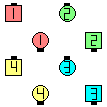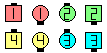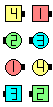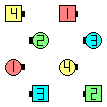Big Block Counter Rotate 1/4\$B\$NA0(B \$BIaDL\$N(B setup \$B\$K=L\$a\$?\$H\$3\$m(B \$B\$N8e(B Counter Rotate 1/4\$B\$N8e(B \$B0LCV\$r0\F0\$7D>\$7\$?\$H\$3\$m(B \$B\$N8e(B (\$B=*\$o\$j(B)

\$B>e\$NNc\$G(B, \$B\$"\$J\$?\$O(B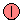\$B\$N?M\$@\$H;W\$C\$F\$/\$@\$5\$\$!%0lHV:8\$N?^\$r8+\$k\$H(B Big Block Counter Rotate 1/4 \$B\$G(B, \$B%3!<%k\$rF0\$/A0\$K(B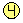\$B\$N?M\$,@j\$a\$F\$\$\$?%]%8%7%g%s\$G=*\$o\$k\$h\$&\$K9M\$(\$k\$3\$H\$,\$"\$j\$^\$9!%(B \$BBig Block Centers Counter Rotate 1/4 \$B\$@\$C\$?\$i\$=\$&\$J\$j\$^\$9\$,(B, \$B\$=\$&\$G\$O\$J\$/(B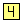\$B\$N?M\$,@j\$a\$F\$\$\$?%]%8%7%g%s\$G=*\$o\$j\$^\$9!%(B
• Formation \$B\$r=L\$a\$F(B, \$B%3!<%k\$r\$7\$F(B, \$B\$b\$H\$XLa\$9\$3\$H\$O>o\$K\$9\$k\$3\$H\$G\$O\$"\$j\$^\$;\$s!%>e\$NNc\$HF1\$8(B formation \$B\$+\$i(B, Big Block Split Counter Rotate 1/4 \$B\$r(B, \$B=L\$a\$FF0\$\$\$FLa\$7\$?\$N\$G\$O\$H\$F\$bF0\$-\$K\$/\$/\$J\$j\$^\$9!%=L\$a\$k\$N\$O(B, \$BJ#;(\$J%3!<%k\$+(B shape-change \$B\$N\$H\$-\$@\$1\$K\$7\$F\$/\$@\$5\$\$!%(B
• \$B1~MQ(B: Big Block anything To A (Normal) Column | Line | Wave: Big Block \$B\$G%3!<%k\$r;O\$a\$^\$9\$,(B, \$BIaDL\$N(B setup (\$BNc\$(\$P(B Parallel Waves) \$B\$G=*\$o\$j\$^\$9!%(B

Stagger Concept [C2]: Stagger \$B\$O(B Interlocked Blocks \$B\$N\$h\$&\$J(B 16-Matrix formation \$B\$+\$i(B, Column \$B\$K\$\$\$k\$H\$7\$F%3!<%k\$r9T\$&\$b\$N\$G\$9!%M?\$(\$i\$l\$?%3!<%k\$r=*\$(\$k\$H\$-\$K(B, \$B3F!9\$N?M\$O%*%j%8%J%k\$N(B formation \$B\$N0LCV\$N0l\$D\$G=*\$o\$i\$J\$1\$l\$P\$J\$j\$^\$;\$s!%(B Shape-changing call \$B\$O(B, \$B3F!9\$N?M\$,%*%j%8%J%k\$N(B formation \$B\$N0LCV\$N0l\$D\$G=*\$o\$k\$3\$H\$K\$D\$\$\$F[#Kf\$5\$,\$J\$\$\$H\$-\$K\$N\$_5v\$5\$l\$^\$9!%(B Interlocked Blocks \$B\$G\$O(B, \$B\$9\$H\$-\$KLrN)\$A\$^\$9!%(BCALLERLAB definition for Big Block ConceptChoreography for Big Block CONCEPTComments? Questions? Suggestions?